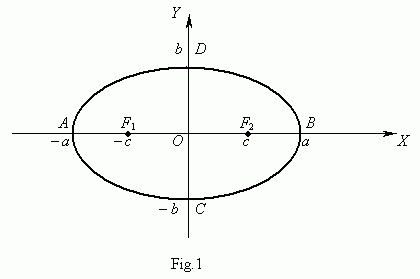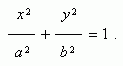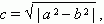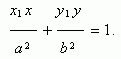Ellipse

Ellipse. Focuses. Equation of ellipse. Focal length.

Large and small axes of ellipse. Eccentricity.

Equation of tangent line to ellipse.

Tangency condition of straight line and ellipse.

An ellipse ( Fig.1 ) is called a locus of points, a sum of distances from which to the two given points F 1 and F 2 , called focuses of ellipse, is a constant value.An equation of ellipse ( Fig.1 ) is :Here the origin of coordinates is a center of symmetry of ellipse, and the coordinate axes are its axes of symmetry. At a > b focuses of ellipse are placed on axis ну ( Fig.1 ), at a < b focuses of ellipse are placed on axis н Y , and at a = b an ellipse becomes a circle ( in this case focuses of ellipse coincide with a center of circle ). Thus, a circle is a particular case of an ellipse . The segment F 1 F 2 = 2 Я , whereis called a focal length . The segment AB = 2 a is called a large axis of ellipse, the segment CD = 2 b is called a small axis of ellipse. The number e = c / a , e < 1 is called an eccentricity of ellipse.

Let п ( У 1 , С 1 ) be a point of ellipse, then an equation of tangent line to ellipse in this point isA tangency condition of a straight line y = m x + k and an ellipse У 2 / a 2 + С 2 / b 2 = 1 :

k 2 = m 2 a 2 + b 2 .